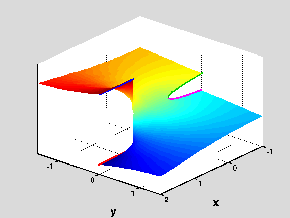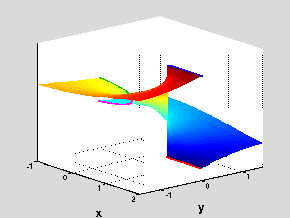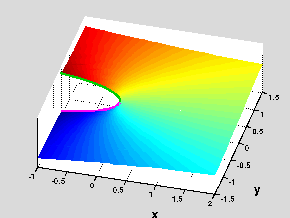18.04 | Fall 1999 | Undergraduate

# Complex Variables with Applications

Study Materials

## Riemann Surfaces: f(z)=log(1-z1/2)

(All images created with MATLAB® software)

Click on pictures for more information and an image of higher resolution.Figure 1: Riemann Surface for the function f(z)=log(1-z1/2)

The Riemann Surface for the function f(z)=log(1-z1/2) has a new feature not seen in any of the prior cases. Namely, in this case the branch points are z=0, z=1 and infinity. However, the branch point at z=1 is peculiar, since it is a branch point only when we take the value of z1/2 as 1 for z=1 and it is not when we take this value to be -1. This means that there are two distinct cut complex planes that one must use in constructing the surface. The first cut plane could be taken as the plane with two cuts on the real axis (on x < 0 and on x > 1) and corresponds to the case when z=1 is a branch point. There are infinitely many branches of the function on this cut plane, with their values differing by multiples of 2πi (depending on which branch of the logarithm one uses). Two views of the resulting typical “Lego” surface block are shown in Figures 1 & 2. To construct the Riemann Surface, we begin by taking infinitely many copies of this “Lego” block, stacking them one on top of the other and joining them across the matching edges of the branch cuts on x > 1 (in the pictures these edges are marked by thick lines in blue and red — the joining is then done blue in one block to red in the next one). After this first step, we end up with a surface that is somewhat similar to the spiral staircase-like Riemann Surface that the logarithm function has, except for a “small” detail: at each level in the staircase, there is a gap left by the two lips of the branch cut on the negative real axis (marked in thick magenta and green lines in the pictures).Figure 2: Riemann Surface for the function f(z)=log(1-z1/2)

To fill the gaps left in the surface after the first construction step described in the prior paragraph, we make use of the second cut plane, which has a single cut (on the negative real axis) and corresponds to the case when z=1 is not a branch point. Again, there are infinitely many branches of the function on this cut plane, with their values differing by multiples of 2πi. A view of the resulting typical “Lego” surface block is shown on Figure 3. We can now use these blocks to fill in the gaps left in the prior step, by joining the appropriate edges of the cuts on the negative real axis (magenta to magenta and green to green) of the two types of “Lego” blocks we have. Notice that this last step can only be done by going into a fourth dimension (to avoid crossings of the surfaces). Thus the Riemann Surface for f(z)=log(1-z1/2) is an object in four dimensional space. We have not attempted to provide a three dimensional projection/view of this surface, but we hope that the description above gives some idea of its structure.Figure 3: Surface generated by one of the branches where z=1 is not a branch point of f(z)=log(1-z1/2).